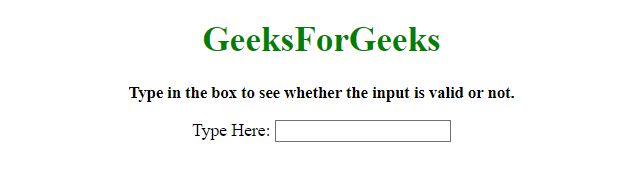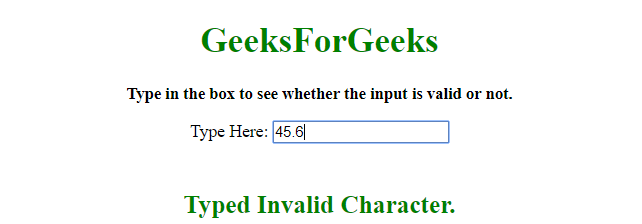# How to restrict input box to allow only numbers and decimal point JavaScript?

The task is to validate the input when the user is typing something in the input box. Here we are allowing only decimal and integers but not any other symbol. Here are a few techniques discussed.

Approach 1:

• A RegExp to verify the input.
• Each time a character is entered, the whole input is matched with the RegExp to check validity.
• If it is valid, make the character valid and add to the input else not.

Example 1: This example using the approach discussed above using JavaScript.

 ` ` `<``html``> ` ` `  `<``head``> ` `    ``<``title``> ` `        ``How to restrict input box to allow ` `      ``only numbers and decimal point JavaScript? ` `    `` ` ` ` ` `  `<``body` `style``=``"text-align:center;"` `      ``id``=``"body"``> ` `   `  `    ``<``h1` `id``=``"h1"` `        ``style``=``"color:green;"``>   ` `            ``GeeksForGeeks   ` `        `` ` `    ``<``p` `id``=``"GFG_UP"`  `       ``style="font-size: 15px;  ` `              ``font-weight: bold;"> ` `    `` ` `    ``<``form``> ` `        ``Type Here: ` `        ``<``input` `id``=``"input"` `               ``oninput``=``"valid(this)"` `               ``type``=``"text"``> ` `    `` ` `    ``<``br``> ` `    ``<``p` `id``=``"GFG_DOWN"`  `       ``style="font-size: 23px; ` `              ``font-weight: bold;  ` `              ``color: green; "> ` `    `` ` `    ``<``script``> ` `        ``var el_up = document.getElementById("GFG_UP"); ` `        ``var el_down = document.getElementById("GFG_DOWN"); ` `        ``el_up.innerHTML =  ` `          ``"Type in the box to see whether the input is valid or not."; ` `        ``var RegExp = new RegExp(/^\d*\.?\d*\$/); ` `        ``var val = document.getElementById("input").value; ` ` `  `        ``function valid(elem) { ` `            ``if (RegExp.test(elem.value)) { ` `                ``val = elem.value; ` `                ``el_down.innerHTML = "Typed Valid Character."; ` `            ``} else { ` `                ``elem.value = val; ` `                ``el_down.innerHTML = "Typed Invalid Character."; ` `            ``} ` `        ``} ` `    `` ` ` ` ` `  ` `

Output:

• Before clicking on the button:• After clicking on the button:Approach 2:

• Each time a character is entered, This character is checked whether this is valid or not.
• This example is also checking the number of decimal entered, one can not enter 2 decimals.
• If the character is valid, add to the input else not.

Example 2: This example using the approach discussed above using JQuery.

 ` ` `<``html``> ` ` `  `<``head``> ` `    ``<``title``> ` `        ``How to restrict input box to allow ` `      ``only numbers and decimal point JavaScript? ` `    `` ` ` ` ` `  `<``body` `style``=``"text-align:center;"` `      ``id``=``"body"``> ` `    ``<``h1` `id``=``"h1"` `        ``style``=``"color:green;"``>   ` `            ``GeeksForGeeks   ` `        `` ` `    ``<``p` `id``=``"GFG_UP"`  `       ``style="font-size: 15px;  ` `              ``font-weight: bold;"> ` `    `` ` `    ``<``form``> ` `        ``Type Here: ` `        ``<``input` `id``=``"input"` `               ``onkeypress``=``"return GFG_Fun(this, event)"`  `               ``type``=``"text"``> ` `    `` ` `    ``<``br``> ` `    ``<``p` `id``=``"GFG_DOWN"`  `       ``style="font-size: 23px;  ` `              ``font-weight: bold;  ` `              ``color: green; "> ` `    `` ` `    ``<``script``> ` `        ``var el_up = document.getElementById("GFG_UP"); ` `        ``var el_down = document.getElementById("GFG_DOWN"); ` `        ``el_up.innerHTML =  ` `          ``"Type in the box to see whether the input is valid or not."; ` ` `  `        ``function isValid(el, evnt) { ` `            ``var charC = (evnt.which) ? evnt.which : evnt.keyCode; ` `            ``if (charC == 46) { ` `                ``if (el.value.indexOf('.') === -1) { ` `                    ``return true; ` `                ``} else { ` `                    ``return false; ` `                ``} ` `            ``} else { ` `                ``if (charC > 31 && (charC < ``48` `|| charC > 57)) ` `                    ``return false; ` `            ``} ` `            ``return true; ` `        ``} ` ` `  `        ``function GFG_Fun(t, evnt) { ` `            ``var a = isValid(t, evnt); ` `            ``if (a) { ` `                ``el_down.innerHTML = "Typed Valid Character."; ` `            ``} else { ` `                ``el_down.innerHTML = "Typed Invalid Character."; ` `            ``} ` `            ``return a; ` `        ``} ` `    `` ` ` ` ` `  ` `

Output:

• Before clicking on the button:• After clicking on the button:My Personal Notes arrow_drop_upCheck out this Author's contributed articles.

If you like GeeksforGeeks and would like to contribute, you can also write an article using contribute.geeksforgeeks.org or mail your article to contribute@geeksforgeeks.org. See your article appearing on the GeeksforGeeks main page and help other Geeks.

Please Improve this article if you find anything incorrect by clicking on the "Improve Article" button below.